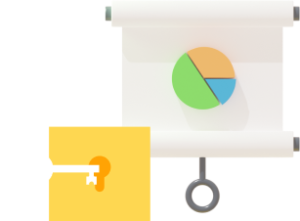# Primary 3 Syllabus

Aligned with the latest 2021 Singapore MOE Math syllabus and endorsed by Education Alliance of Finland.
Hundreds of hours of learning content for all topics covered in P3 to get your child build a strong Math foundation!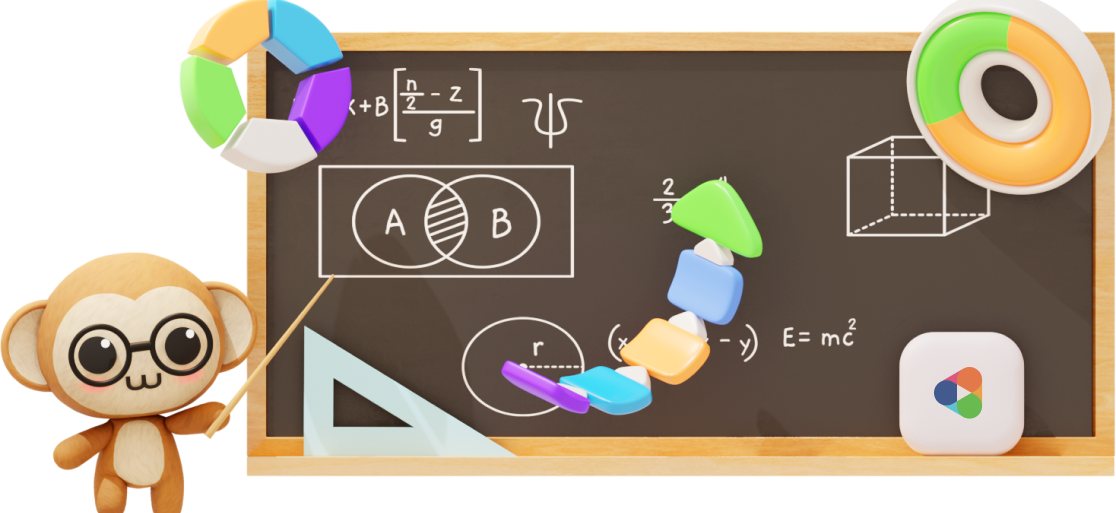## Overview

In P3, your child will expand their problem-solving toolbox by learning heuristics, such as drawing diagrams and looking for patterns.

If your child’s foundation is weak, they will struggle. Soon, they dread doing their homework or going to class.

They are afraid that they can’t keep up.

With Practicle, they can!

First, our platform will use AI to personalise our questions to match your child’s current ability.

Then, over time, as your child improves, we’ll give them tougher questions.

Even if the class has moved on, they can continue to build their skills.

That’s the beauty of self-paced learning.

Our syllabus is 100% aligned with MOE and is certified by the Education Alliance of Finland (EAF) for educational quality and effectiveness.

### Features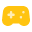##### Gamification to make learning engaging and rewarding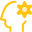##### A.I. + Practicle system to personalise questions to your child’s ability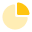### Primary 3 Math Syllabus – What’s in it

Practicle’s Math questions cover all topics and skills in the Singapore Primary 3 Math curriculum. Here’s a list of all the P3 topics and skills that are tested in our multiple-choice questions (MCQs) and word problem sums.

The 2021 Primary 3 Math Syllabus can be divided into 3 main branches – Numbers, Measurement and Geometry and finally, Graphs. In each branch, the Math questions will be divided into 3 difficulty levels – easy, medium and hard.

#### P3 Math: Numbers

The Primary 3 (P3) topics that are covered under Numbers are Whole Numbers, Addition, Subtraction, Multiplication, Division, Fractions and Money. Here’s the breakdown of the skills in each topic:#### Whole Numbers

• Count in thousands
• Compare and order numbers
• Determine the place value of a number up to 1 000
• Complete number patterns
• Solve word problems with 4 operations#### Subtraction

• Subtract 4 digit numbers
• Solve subtraction word problems#### Multiplication

• 6 Multiplication table
• 7 Multiplication table
• 8 Multiplication table
• 9 Multiplication table
• Multiply up to 3 digit by 1 digit
• Solve multiplication word problems#### Division

• Divide by 6
• Divide by 7
• Divide by 8
• Divide by 9
• Divide with remainders
• Divide up to 3 digits by 1 digit
• Solve division word problems#### Fractions

• Simplify a fraction
• Compare and order fractions
• Write equivalent fractions
• Subtract 2 related fractions#### Money

• Subtract money in decimals
• Solve money word problems

### P3 Math: Measurement and Geometry

The Primary 3 (P3) topics that are covered under Measurement and Geometry are Length, Mass, Volume, Time, Angles, Perpendicular and Parallel Lines as well as Area and Perimeter. See below for the breakdown of skills in each topic:#### Length

• Measure length in km
• Measure length in compound units
• Convert km and m to m and vice versa
• Convert m and cm to cm and vice versa
• Solve length word problems#### Mass

• Measuring length in centimetres
• Comparing and ordering length
• Curves and straight lines#### Volume

• Measure volume in ml
• Measure volume in compound units
• Convert l and ml to ml and vice versa
• Solve volume word problems#### Time

• Tell time to the minute
• Use “past” and “to” in time
• Calculate time in hours and minutes
• Convert time from hours and minutes to minutes and vice versa
• Solve time word problems#### Lines

• Identify perpendicular lines
• Identify parallel lines
• Identify horizontal and vertical lines#### Angles

• Identify right angles
• Identify acute angles
• Identify obtuse angles#### Area & Perimeter

• Find area and perimeter of a plane figure
• Find area in square units
• Find the perimeter of a rectilinear figure
• Find perimeter of square
• Find perimeter of rectangle
• Find the area of a square
• Find the area of a rectangle

### P3 Math: Graphs

The Primary 3 (P3) topics that are covered under Graphs include data analysis of bar graphs. Refer below for the breakdown of the skills in this topic.### Sample P3 questions and video explanations### Experience Practicle free, no commitment no hidden cost no lock-in

Try our learning platform free with a 7 day trial
and see if your child likes it.